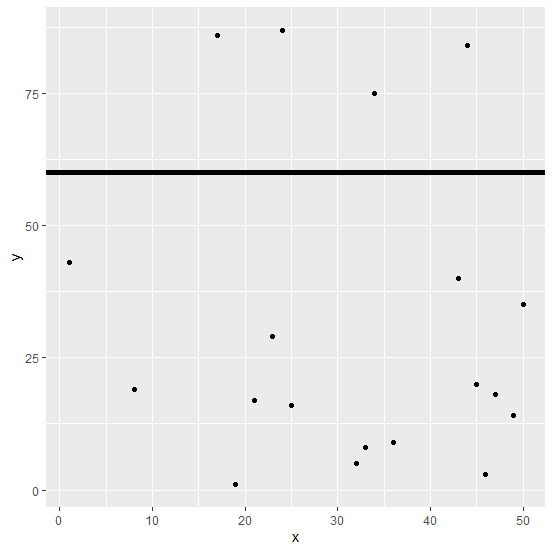# How to create a horizontal line in ggplot2 graph with larger width in R?

To create a horizontal line in ggplot2 graph with larger width in R, we can follow the below steps −

• First of all, create a data frame.
• Then, create a plot using ggplot2.
• After that, create the same plot with geom_hline function having horizontal line defined with yintercept and its width defined with size argument

## Create the data frame

Let's create a data frame as shown below −

Live Demo

> x<-sample(1:50,20)
> y<-sample(1:100,20)
> df<-data.frame(x,y)
> df

On executing, the above script generates the below output(this output will vary on your system due to randomization) −

    x y
1   1 43
2  21 17
3  36 9
4  43 40
5   8 19
6  34 75
7  23 29
8  44 84
9  33 8
10 24 87
11 45 20
12 17 86
13 9 60
14 50 35
15 46 3
16 49 14
17 47 18
18 19 1
19 25 16
20 32 5

## Create the plot using ggplot2

Let’s create a scatterplot between x and y −

> x<-sample(1:50,20)
> y<-sample(1:100,20)
> df<-data.frame(x,y)
> library(ggplot2)
> ggplot(df,aes(x,y))+geom_point()

## Output## Create the plot with wider horizontal line

Using geom_hline to create the horizontal line in the above plot with yintercept = 60 and size = 2 −

> x<-sample(1:50,20)
> y<-sample(1:100,20)
> df<-data.frame(x,y)
> library(ggplot2)
> ggplot(df,aes(x,y))+geom_point()+geom_hline(yintercept=60,size=2)

## Output